Please check for Class 12 - Biology Important Questions

### List of Questions and Answers

Q 1.What type of liquids form ideal solutions?

Ans:
The solution is said to be an ideal solution when two liquids A and B on mixing has their intermolecular forces of attraction between A – A, B – B and A – B are nearly equal.

Q 2.What happens when blood cells are placed in pure water?

Ans:
When blood cells are placed in water ,due to osmosis, water molecules moves into the blood cells through the cell walls. As a result the blood cells swells and can also burst.

Q 3.Define the term solution. How many types of solutions are formed? Write briefly about each type with an example.

Ans:
Solutions are homogeneous mixtures of two or more than two components. Homogeneous refers to a mixture where its compositions and properties are uniform throughout. The component which is present in larger quantity is said to be solvent and other components which are present are called solute. For example salt water, here water is solvent and salt is solute. So the final equation Solvent + Solute = Solution. Please note the component ie solvent or solute can be in solid, liquid or gaseous in nature.
There can be 9 types of such combination.Here is the list of them along with examples:

Type of Solution Solute Solvent Examples
Gaseous Solutions Gas Gas Mixture of oxygen and nitrogen gases
Liquid Gas Chloroform mixed with nitrogen gas
Solid Gas Camphor in nitrogen gas
Liquid Solutions Gas Liquid Oxygen dissolved in water
Liquid Liquid Ethanol dissolved in water
Solid Liquid Glucose dissolved in water
Solid Solutions Gas Solid Solution of hydrogen in palladium
Liquid Solid Amalgam of mercury with sodium
Solid Solid Copper dissolved in gold

In the above chart we have seen solutions can be of 3 types , Gaseous, Liquid and Solid Solutions.
Gaseous Solutions : In gaseous solutions the solvent will be gas and the solute can be solid, gas or liquid.For example, a mixture of oxygen and nitrogen gas is a gaseous solution.
Liquid Solutions : In liquid solutions the solvent will be liquid and the solute can be solid , gas or liquid.For example Glucose dissolved in water.
Solid Solutions : In case of solid solutions the solvent will be solid and solute can be gas, liquid or solid.For example copper dissolved in gold.

Q 4.Calculate the mole fraction of ethylene glycol (C 2H 6O 2) in a solution containing 20% of C 2H 6O 2 by mass.

Ans:
Assume that we have 100 g of solution (one can start with any amount of solution because the results obtained will be the same). Solution will contain 20g of ethylene glycol and 80g of water.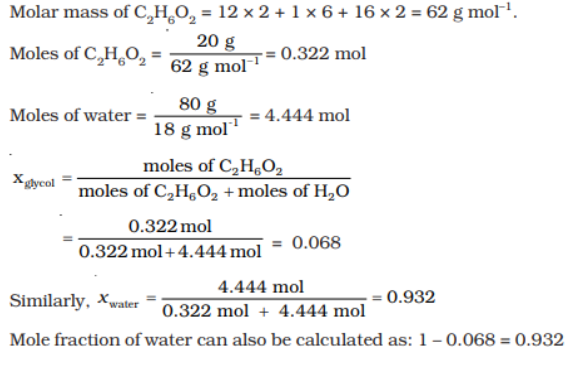Q 5.Calculate the molarity of a solution containing 5 g of NaOH in 450 mL solution.

Ans: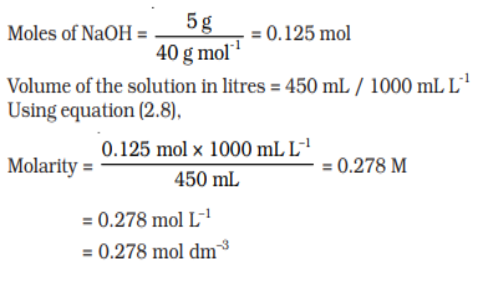Q 6.Give an example of “liquid in solid‘ type solution.

Ans:
In such case the solvent is solid and solute is liquid.Example is Amalgam of mercury with sodium.

Q 7.Give an example of “solid in liquid” type solution.

Ans:
In such case solvent is liquid and solute is solid.Example is Glucose dissolved in water.

Q 8.Give an example of “gas in liquid” type solution.

Ans:
In such case solvent is liquid and solute is gas.Example is Oxygen dissolved in water.

Q 9.Why it is advised to add ethylene glycol to water in a car radiator while driving in a hill station?

Ans:
Ethylene glycol is used as a antifreeze in cars.As ethylene glycol has low freezing point when added to water it can resists the freezing when the temperature gets low specially when travelling to hill station.

Q 10.Why do gases nearly always tend to be less soluble in liquids as the temperature is raised?

Ans:
The dissolution of gas in a liquid is said to be exothermic reaction.As per Le Chatelier's principle, when the temperature increases , the equilibrium shifts in the backward direction which means the solution will dissociate and will give gas. Gas + Liquid → Solution + Heat Therefore the solubility of gas in solution decreases with rise in temperature.

Q 11.Explain dissolution and crystallisation.

Ans:
When a solid solute is added to the solvent, some solute dissolves and its concentration increases in solution. This process is known as dissolution. Some solute particles in solution collide with the solid solute particles and get separated out of solution. This process is known as crystallisation. A stage is reached when the two processes occur at the same rate. Under such conditions, number of solute particles going into solution will be equal to the solute particles separating out and a state of dynamic equilibrium is reached.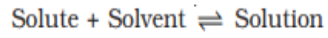Q 12.If N 2 gas is bubbled through water at 293K, how many millimoles of N 2 gas would dissolve in 1 litre of water. Assume that N 2 exerts a partial pressure of 0.987 bar. Given that Henry’s law constant for N 2 at 293 K is 76.48 kbar.

Ans:
The solubility of gas is related to the mole fraction in aqueous solution. The mole fraction of the gas in the solution is calculated by applying Henry’s law. Thus: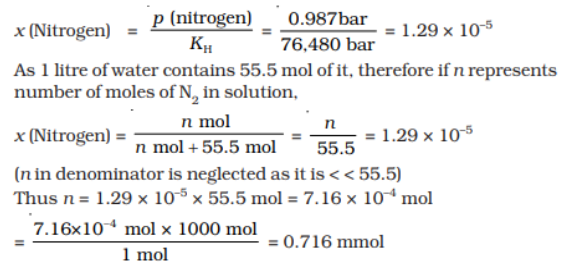Q 13.H 2S, a toxic gas with rotten egg like smell, is used for the qualitative analysis. If the solubility of H 2S in water at STP is 0.195 m, calculate Henry's law constant.

Ans:
It is given that the solubility of H2S in water at STP is 0.195 m, i.e., 0.195 mol of H 2S is dissolved in 1000 g of water.
Moles of water = 1000 g / 18 g mol-1
= 55.56 mol
∴Mole fraction of H 2S, x = Moles of H 2S / Moles of H 2S + Moles of water
= 0.195 / (0.195+55.56)
= 0.0035
At STP, pressure (p) = 0.987 bar
According to Henry's law:
p = KHx
KH = p / x
= 0.0987 / 0.0035 bar
= 282 bar

Q 14.Henry’s law constant for CO 2 in water is 1.67 × 10 8 Pa at 298 K. Calculate the quantity of CO 2 in 500 mL of soda water when packed under 2.5 atm CO 2 pressure at 298 K.

Ans:
Pressure of CO 2 = 2.5 atm
1 atm = 1.01325 × 10 5 Pa , So Pressure of CO 2 = 2.5 × 1.01325 × 10 5 Pa = 2.533125 × 10 5 Pa
K H = 1.67 × 10 8 Pa
According to Henry’s law:
p = K H × X
X = p / K H = 2.533125 × 10 5 / 1.67 × 10 8 = 1.52 x 10 –3
We have 500 mL of soda water so that
Volume of water = 500 mL
Density of water = 1 g/ ml
mass = volume × density
500 mL of water = 500 g of water
Molar mass of water(H 2O) = 18 g mol -1 water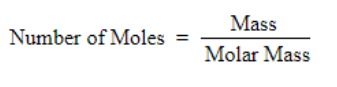Number of mole of water = 500 / 18 = 27.78 mol of water
Formula of mole fraction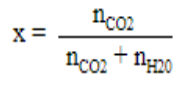Value of mole fraction is very small so it is negligible as compared to 1
We get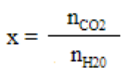1.52 × 10 –3 = n CO 2 / 27.78
n CO 2 = 0.042 mol
molar mass of CO 2 = 12 + 2 × 16 = 44 g mol -1
mass = molar mass × number of moles
= (0.042 × 44)g
= 1.848 g

Q 15.Calculate molality of 2.5 g of ethanoic acid (CH 3COOH) in 75 g of benzene.

Ans:
Molar mass of C 2H 4O 2: 12 × 2 + 1 × 4 + 16 × 2 = 60 g mol –1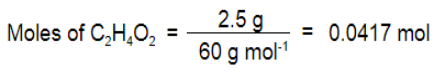Mass of benzene in kg = 75 g/1000 g kg –1 = 75 × 10 –3 kg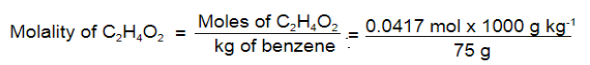= 0.556 mol kg –1

Q 16.Why are soft drink and soda bottles sealed under high pressure?

Ans:
To increase the solubility of carbon dioxide in soft-drinks and soda bottles , the bottles are sealed under high pressure.If the pressure is not maintained, CO2 will come out and escape in the atmosphere leaving just the water solution.

Q 17.Why are tanks used by scuba divers filled with air diluted with helium?

Ans:
Scuba divers when they go underwater have to breathe air dissolved with high concentration gaseous which are mixed with blood.When they reach the surface the pressure decreases , which causes the gases to release and leads to bubbles of nitrogen in the blood.This blocks the capillaries and causes a medical condition called blends which is very dangerous for life.To reduce this condition the tanks are filled with air diluted with helium(11.7% helium, 56.2% nitrogen and 32.1% oxygen).

Q 18.Why helium is used in tanks used by scuba divers?

Ans:
Helium is less soluble in water and because of its low solubility it does not get mixed with blood when the pressure changes.Scuba divers has to go underwater where the pressure is high and they come to the surface the pressure is changed.Helium is a inert gas which is required to dilute oxygen to handle the pressure change as high concentration of oxygen in blood can cause health issues.

Q 19.The vapour pressure of pure benzene at a certain temperature is 0.850 bar. A non-volatile, non-electrolyte solid weighing 0.5 g when added to 39.0 g of benzene (molar mass 78 g mol-1). Vapour pressure of the solution, then, is 0.845 bar. What is the molar mass of the solid substance?

Ans: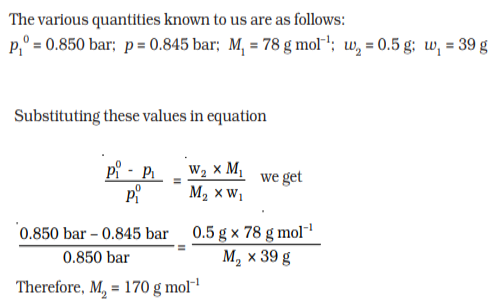Q 20.State Henry’s law and mention some important applications?

Ans:
The law states that at a constant temperature, the solubility of a gas in a liquid is directly proportional to the pressure of the gas. Important Applications of Henry’s Law:
To increase the solubility of CO2 in soft drinks and soda water, the bottle is sealed under high pressure.
Helium is added to tanks used by scuba divers so that the gas is not mixed under high pressure.
At high altitudes the partial pressure of oxygen is less than that at the ground level. This leads to low concentrations of oxygen in the blood and tissues of people living at high altitudes or climbers. Low blood oxygen causes climbers to become weak and unable to think clearly, symptoms of a condition known as anoxia.

Q 21.Aquatic animals are more comfortable in cold water than in warm water.

Ans :
The amount of oxygen soluble in water increases with decrease of temperature and it is less when the temperature rises , due to which aquatic species are more comfortable in cold waters rather than in warm waters.

Q 22.State Raoult’s law.

Ans:
It states that for a solution of volatile liquids, the partial vapour pressure of each component in the solution is directly proportional to its mole fraction.

Q 23.Explain why on addition of 1 mol of NaCl to 1 litre of water, the boiling point of water increases.

Ans:
NaCl is a non-volatile solute.When a non volatile solute is added to a solvent, its vapour pressure decreases because some of the surface sites are occupied by solute molecules.Thus, less space is available for the solvent molecules to vaporise.Hence boiling point of water increases.

Q 24.What do you understand by depression of freezing point?

Ans:
Depression of freezing point is the decrease of the freezing point of a solvent on addition of a non-volatile solute. Examples include salt in water, alcohol in water.

Q 25.On mixing liquid X and liquid Y, the volume of the resulting solution increases. What type of deviation from Raoult’s law is shown by the resulting solution ?What change in temperature would you observe after mixing liquids X and Y ?

Ans:
Negative deviation is shown by Raoult’s law.The boiling point of the solution increases which in-turn increases the temperature.

Q 26.What is meant by positive and negative deviation from Raoult’s law ? Give an example.

Ans:
When solutions does not obey Raoult’s law over their entire range of concentration they are called non-ideal solutions.If the vapour pressure of such solution is higher than it exhibits positive deviation and if the vapour pressure is less it exhibits negative deviation.
In case of positive deviation from Raoult’s law, A-B interactions are weaker than those between A-A or B-B, i.e., in this case the intermolecular attractive forces between the solute-solvent molecules are weaker than those between the solute-solute and solvent-solvent molecules.This means that in such solutions, molecules of A (or B) will find it easier to escape than in pure state.This will increase the vapour pressure and result in positive deviation. Example mixtures of ethanol and acetone behave in this manner.
In case of negative deviations from Raoult’s law, the intermolecular attractive forces between A-A and B-B are weaker than those between A-B and leads to decrease in vapour pressure resulting in negative deviations. An example of this type is a mixture of phenol and aniline.

Q 27.Define osmotic pressure of a solution. How is the osmotic pressure related to the concentration of a solute in a solution ?

Osmotic pressure is the minimum pressure which needs to be applied to a solution to prevent the inward flow of its pure solvent across a semipermeable membrane.
Osmotic pressure is proportional to the molarity, C of the solution at a given temperature T. Therefore,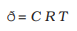Here ð is the osmotic pressure and R is the gas constant.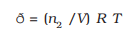Here V is volume of a solution in litres containing n 2 moles of solute.
If w 2 grams of solute, of molar mass, M 2 is present in the solution, then n 2 = w 2 / M 2 and we can write,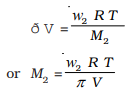Thus, knowing the quantities w 2, T,ð and V we can calculate the molar mass of the solute.

Q 28.A solution is prepared by dissolving 5g of non-volatile solute in 95g of water.It has a vapour pressure of 23.375 mm Hg at 25 o C. Calculate the molar mass of the solute. (Vapour pressure of pure water at 25 o C is 23.75 mm Hg)

Ans:
According to Raoult’s Law: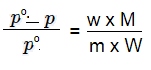p0 -- Vapour Pressure of Pure Water
p -- Vapour Pressure of Solvent
w -- weight of solute
m -- molecular weight of solute
W -- molecular weight of Solvent
M -- Molar Mass of Water = 18 g mol -1
Substituting the values in the formula given above we get foll :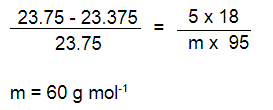Q 29.Calculate the freezing point of an aqueous solution containing 10.5g of Magnesium bromide in 200g of water , assuming complete dissociation of Magnesium bromide.
(Molar mass of Magnesium bromide = 184 g mol -1 , Kf for water = 1.86 K kg mol -1).

Ans: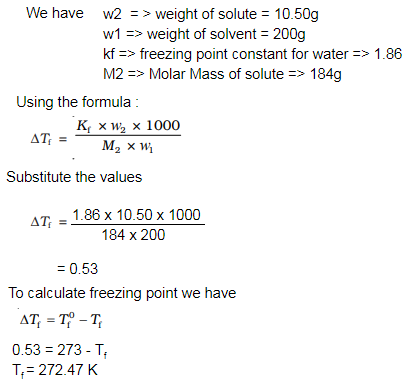Q 30.Calculate the boiling point elevation for a solution prepared by adding 10g CaCl2 to 200g of water, assuming that CaCl2 is completely dissociated.(Kb for water = 0.512K Kg mol -1; Molar mass of CaCl 2 = 111 g mol -1)

Ans: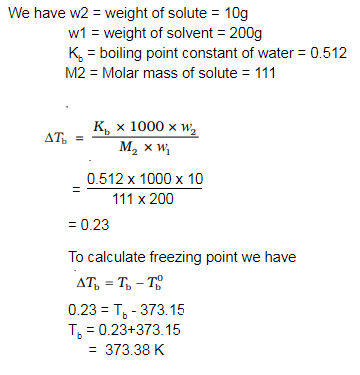Q 31.Define following terms
i) Colligative properties
ii) Azeotrope

Ans:
Colligative properties of solutions are properties that depend upon the concentration of solute molecules irrespective of their nature relative to the total number of particles present in the solution.Colligative properties include vapor pressure lowering, boiling point elevation, freezing point depression, and osmotic pressure.
An azeotrope is a binary mixture of liquids that maintains same composition in liquid and vapour phase and boil at a constant temperature.

Q 32.Define the following terms :
(i) Molarity
(ii) Mole Fraction
(iii) Molal elevation constant (Kb)

Ans:
(i) Molarity: Molarity (M) is defined as number of moles of solute dissolved in one litre (or one cubic decimetre) of solution.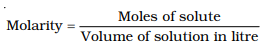(ii) Mole fraction is a unit of concentration, defined to be equal to the number of moles of a component divided by the total number of moles of a solution.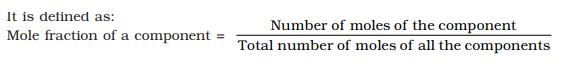(iii) Molal elevation constant (Kb) :The number of moles of solute dissolved in 1 kg of solvent and the constant of proportionality, Kb is called Boiling Point Elevation Constant or Molal Elevation Constant (Ebullioscopic Constant). The unit of Kb is K kg mol -1.